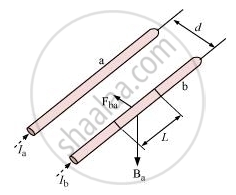Advertisement Remove all ads

# Derive the Expression for Force per Unit Length Between Two Long Straight Parallel Current Carrying Conductors. Hence Define One Ampere. - Physics

Advertisement Remove all ads
Advertisement Remove all ads
Advertisement Remove all ads

Derive the expression for force per unit length between two long straight parallel current carrying conductors. Hence define one ampere.

Advertisement Remove all ads

#### Solution

Two long parallel conductors a and b separated by a distance l and carrying currents Ia and Ib respectively are shown below.By Ampere’s circuital law, we have

B_a = (mu_0I_a)/(2pid)

Conductor b will experience a sideways force because of conductor a. Let this force be Fba.

F_(b_a) = I_bLB_a          (F =ILB)

= (mu_0I_aI_bL)/(2pid)

By symmetry,

Fba = − Fab

1 ampere is the value of that steady current which when maintained in each of the two very long, straight, parallel conductors of negligible cross section and placed one metre apart in vacuum, would produce on each of these conductors a force equal to 2 × 10−7 Newton per metre of length.

Concept: Force Between Two Parallel Currents, the Ampere
Is there an error in this question or solution?

#### Video TutorialsVIEW ALL 

Advertisement Remove all ads
Share
Notifications

View all notifications

Forgot password?